# Transient Magnetic: solved equations (introduction)

## Introduction

The equations used for the solving are:

• Maxwell's equations (for a magnetic system)
• the constitutive equations of the matter

The computation conditions for a Transient Magnetic application are the following:

• the state variables are time dependent: d/dt ≠ 0 (transient or variable state: current sources vary with time)
• the computation concerns only the B, H and E fields (the D field is not computed). The equations of the electric fields E and D and of the magnetic fields B, H can't be decoupled.

## Equations and conditions

In the previously defined conditions of computation, the equations are summarized as follows: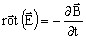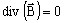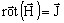E : electric field strength (in V/m) B: magnetic flux density (in T) H: magnetic field strength (in A/m) J: current density (in A/m2)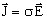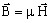σ : conductivity (in S) μ : permeability (in H/m)

The principal equation for magnetic materials, can be put in form B(H) or H(B) as presented below.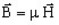⇒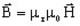μr : perméabilité relative μ0 : perméabilité du vide Br : induction rémanente (aimants)
ou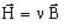⇒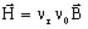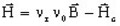νr : reluctivity νr =1/μr ν0 : vacuum reluctivity ν0 =1/μ0 Hc : coercive magnetic field (permanent magnets)

## Models

To solve these equations, two models are used:

• the vector model, which uses:

the magnetic vector potential (written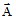)

• the scalar model, which uses:

magnetic scalar potentials (written ϕtot or ϕred)

## Model and 2D or 3D application

For 2D applications, the vector model is available by default.

For 3D applications, the scalar model is available by default.